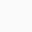# What is a Decimal?

Anything right side of decimal point is decimal numbers. Watch the figure. It is used to represent the fraction of a whole number. Decimal point separate the whole number and decimal number. Decimal provide the ease of writing whole number and decimal number in one number.

If it wouldn’t be there then we would have never been acurate while doing precision measurement ie fine measurement. Our science would have failed at every steps. Therefore decimal number is one that give great solution to science and mathematics.

The decimal number given in the figure is read as six point three eightSee this figure to gain the concept of various form of a number.

# Watch this video

##### One thought on “What is a Decimal?”
1.Azra Ahmad says:

This post be corrected.